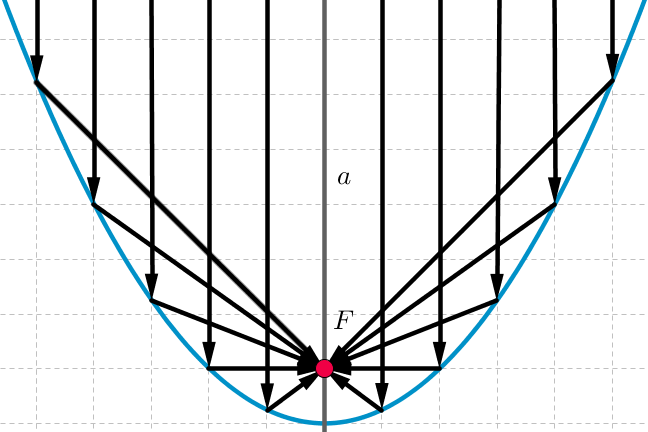## Want to keep learning?

This content is taken from the UNSW Sydney's online course, Maths for Humans: Linear, Quadratic & Inverse Relations. Join the course to learn more.
2.30

## UNSW SydneyParabolic mirror

# Summary and Discussion of quadratic relations

Here is a brief summary of this week.

We began with a historical overview of how the ancient Greeks thought about conic sections, and some of the many properties they discovered about ellipses, parabolas and hyperbolas. Descartes realised that algebraically these were exactly the second degree equations in $$\normalsize{x}$$ and $$\normalsize{y}$$. In particular we had a good look at the parabola and its properties, since this is the geometrical curve that corresponds to a general quadratic function of the form $$\normalsize{y=ax^2+bx+c}$$.

Then we studied a variety of quadratic relations, including area, kinetic energy, motion of a ball thrown vertically and trajectories of projectiles, along with applications in economics.

The algebraic side of quadratic equations centres on the remarkable identity of al Khwarizmi obtained by completing the square, and the associated celebrated quadratic formula. This allows us to understand zeroes of quadratics, and connects with Descartes theorem on factors.

The shape and position of a general quadratic was looked at. Hopefully you have a good sense of how to analyse a given quadratic function, say something like $$\normalsize{y=2x^2-3x+7}$$. We were able to exploit this algebraic understanding to find vertices, evaluate maxima and minima of such curves, and even to obtain formulas for secant and tangent lines, all without any calculus.

We saw how parabolas figure prominently in modern design and architecture, including the Sydney Harbour bridge, along with the remarkable de Casteljau Bezier curves. These were based on the interesting idea of specifying curves by parameters, and we started by examining how to parametrise line segments, and then quadratic curves, and had some pictures of cubic curves too.

And we looked at the algebraic problem of fitting a quadratic function to three specified points, which touched base with the useful technique of solving a system of three equations in three unknowns, going back to the ancient Chinese. We have covered a lot of territory, well done!

## Discussion

This is an opportunity to make comments and ask questions of a general nature about this week’s material. Then there is a test, and then on to Week 3 and inverse relations, where the hyperbola takes the lead role.

Activity image credits: “Conic sections” © Magister Mathematicae/Wikimedia Commons CC BY SA 3.0; “Circular Paraboloid” © Sam Derbyshire/Wikimedia Commons CC BY SA 3.0; “Thermometer with Fahrenheit and Celsius” Public Domain/Wikimedia Commons; “Discussion” © Muhammad Rafizeldi/Wikimedia Commons CC BY SA